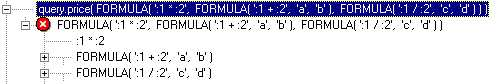FORMULA()

In method call syntax (i.e. in places where SQL syntax is not expected), the FORMULA() application method subjects values to operations that require SQL evaluation.

Use FORMULA() outside SQL, i.e. in method call syntax, to:

 · Subject values to scalar SQL functions such as ROUND(), SUBSTR() and so on.

 · Subject numerical values to complex mathematical operations.

 · Concatenate string values.

The FORMULA() method is an embedded method call that returns a string value to the embedding method call. It takes string parameters that are either surrounded by single quotes, or represented by embedded method calls returning a string value.

You can use any SQL syntax supported by your database vendor. To keep your USoft application portable between databases, however, you should adhere to standards. This is particularly a concern when calling SQL functions. USoft converts certain key SQL functions automatically between databases. Others are database-specific. Please refer to USoft Definer Help for details.

In the Object Activator, a special Formula button helps you write FORMULA() expressions more easily.

Other ways of using SQL where method calls are expected are to call the SqlScript() method, or to call ActionDecision() and retrieve data in the Decision SQL. Use SqlScript() to manipulate data in database tables, and Decisions to retrieve data from database tables.

Example 1

FORMULA(':1 || :2',query.file_name(),'.TXT')

This example reads an on-screen file name value currently held by a base column control, and concatenates it with a .TXT file name extension. The file name is a string value returned by an embedded method call. The file extension is a hard-coded string.

The first parameter represents the SQL expression in which one or more values take part. The second, third, etc. parameters represent the values themselves. The first parameter uses placeholders (:1, :2 and so on) to position the values within the SQL expression.

Example 2

FORMULA(':1 || SUBSTR(:2,INSTR(:2,:3)+1)',

'Domain = ', query.email(), '@')

This example reads an on-screen email address value currently held by a base column control, strips it down to the domain name (the part of the address after the '@' symbol), and prefixes it with the text "Domain =". The resulting return value could be used in a message as in:

MessageInformation

(

FORMULA(':1 || SUBSTR(:2,INSTR(:2,:3)+1)',

'Domain = ', query.email(), '@')

)

Example 3

MessageInformation

(

FORMULA(':1 || :2',

'Total salary for this department is ', :1)

)

This example shows a Yes Action in a decision, and illustrates the combined use of decision placeholders and FORMULA() placeholders. The :1 placeholder at the end of the statement is a decision placeholder. The value it represents is retrieved by the Decision SQL statement, which in this case could read:

SELECT  sum(sal)

FROM    emp

WHERE   deptno = :DEPTNO

The :1 and :2 placeholders in the first parameter of FORMULA() represent the hard-coded message and the sum of salaries, respectively. FORMULA() placeholders can only appear in the first parameter of a FORMULA() call.

Example 4

QueryDefine(  )

query.start_date( FORMULA( ''>'||TO_CHAR(TO_DATE(:1,'YYYY/MMDDHH24MISS'),'DD-MM-YYYY')',  myStartDate() ) )

query.return_date( FORMULA( ''<'||TO_CHAR(TO_DATE(:1,'YYYY/MMDDHH24MISS')+:2,'DD-MM-YYYY')',  myStartDate(),  myNumberOfDays() ) )

QueryExecute(  )

This example shows the use of FORMULA to calculate a date, and illustrates some guidelines to calculate a date using FORMULA:

 · Explicitly use TO_DATE to format the date in USoft's standard IO format, then calculate the date using an argument

 · Afterwards, use TO_CHAR to format the date back as you wish

Refer to Action Example 9 to view this example in its full context.

Example 5

query.price( FORMULA( ':1 * :2',  FORMULA( ':1 + :2',  'a',  'b' ),  FORMULA( ':1 / :2',  'c',  'd' ) ) )

This example represents (a+b) * (c/d) calculation. It illustrates the advantage of using FORMULA which is its clear tree-like structure rather than a long nested line of code. This tree-like structure is also clearly shown when you write the FORMULA in the Object Activator as follows: